## 15.1 高效的数据表示

• 40, 27, 25, 36, 81, 57, 10, 73, 19, 68
• 50, 25, 76, 38, 19, 58, 29, 88, 44, 22, 11, 34, 17, 52, 26, 13, 40, 20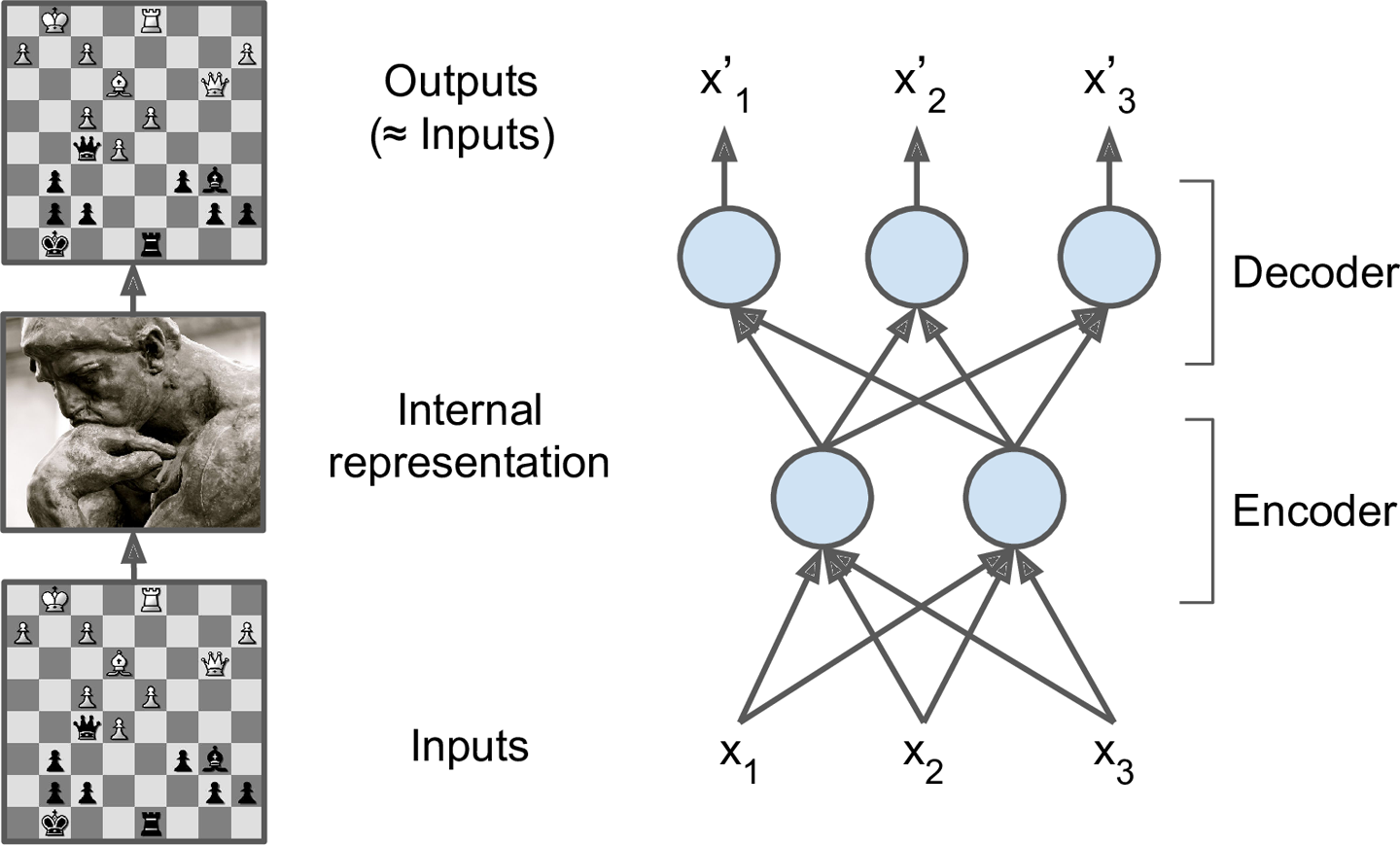undercomplete应该是个数学概率，不用深究了，毕竟在Wikipedia上面的解释只有一句话Describing a frame (in linear algebra) having a set of functions less than a basis

## 15.2 不完备线性自编码器实现PCA（Performing PCA with an Undercomplete Linear Autoencoder）

import tensorflow as tf
from tensorflow.contrib.layers import fully_connected

n_inputs = 3 # 3D inputs
n_hidden = 2 # 2D codings
n_outputs = n_inputs

learning_rate = 0.01

X = tf.placeholder(tf.float32, shape=[None, n_inputs])
hidden = fully_connected(X, n_hidden, activation_fn=None)
outputs = fully_connected(hidden, n_outputs, activation_fn=None)

reconstruction_loss = tf.reduce_mean(tf.square(outputs - X)) # MSE

training_op = optimizer.minimize(reconstruction_loss)

init = tf.global_variables_initializer()

X_train, X_test = [...] # load the dataset

n_iterations = 1000
codings = hidden # the output of the hidden layer provides the codings

with tf.Session() as sess:
init.run()
for iteration in range(n_iterations):
training_op.run(feed_dict={X: X_train}) # no labels (unsupervised)
codings_val = codings.eval(feed_dict={X: X_test})


## 15.3 栈式自编码器（Stacked Autoencoders）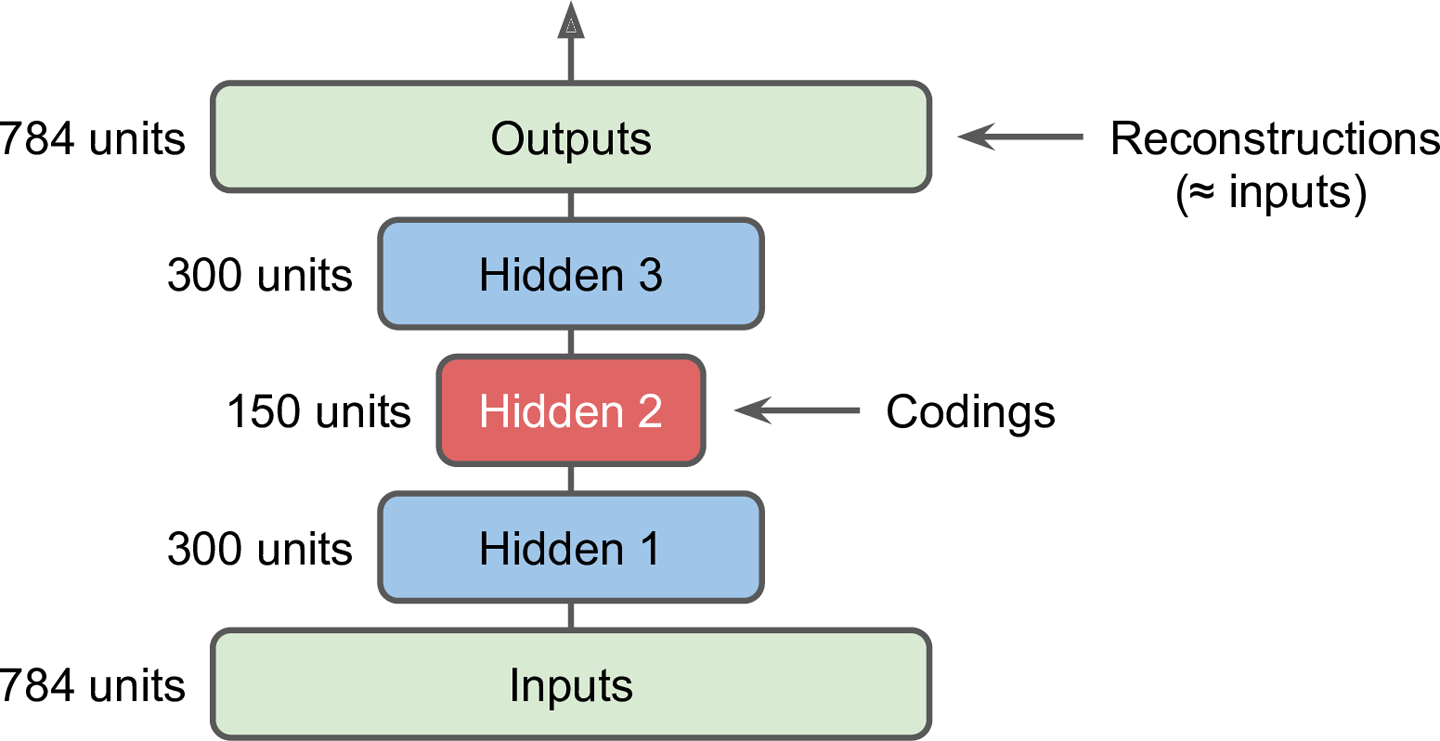### 15.3.3 一次训练一个自编码器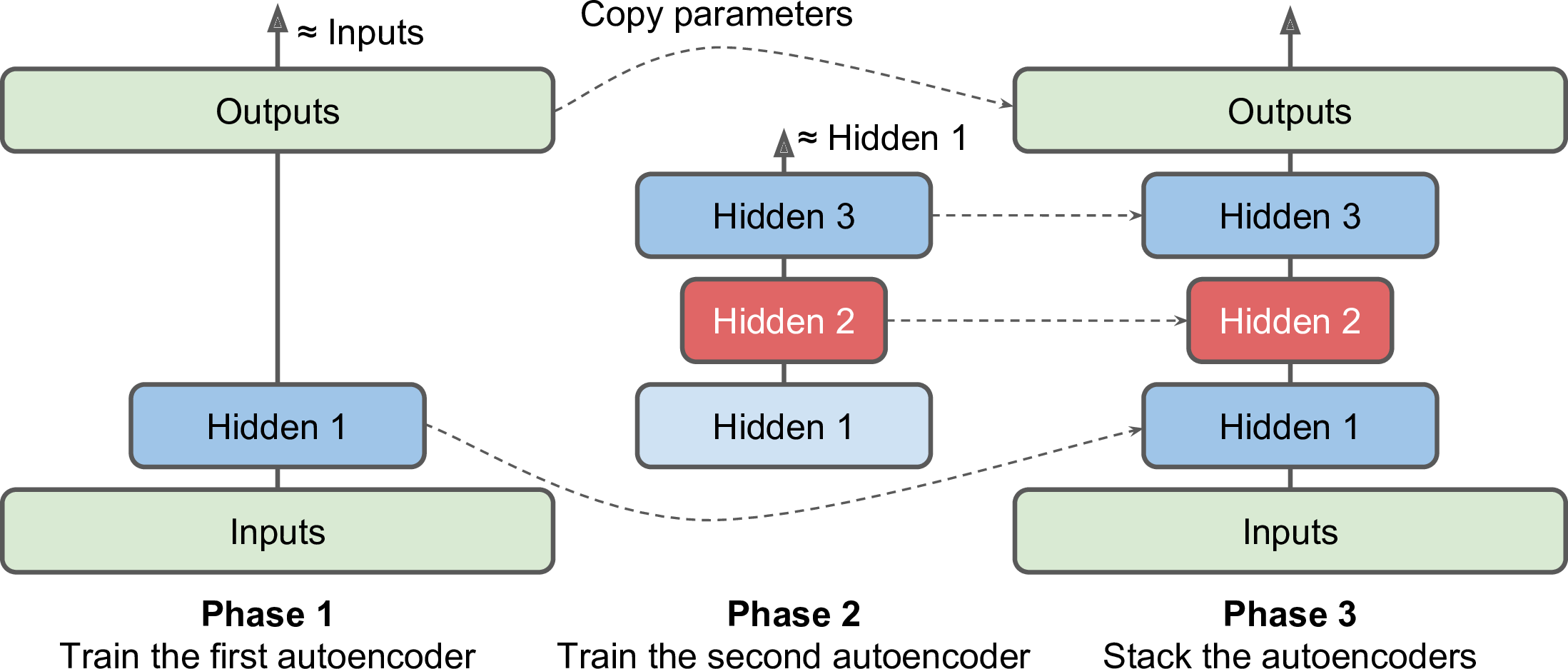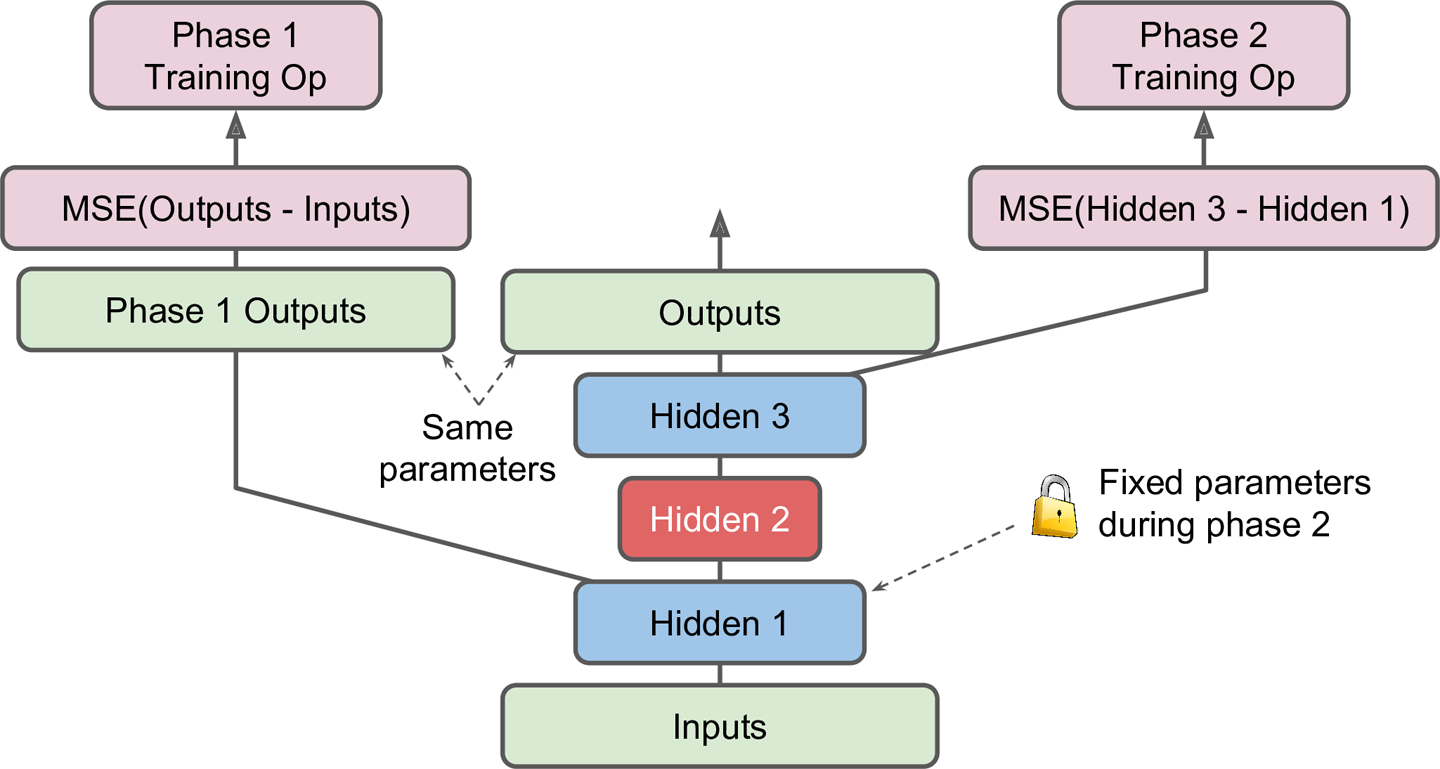• 中间的一列是完整的栈式编码器，这部分在训练完成之后可以使用。
• 左侧一列是最先需要训练的，它跳过第二和第三个隐层，直接创建一个输出层。这个输出层与栈式自编码器的输出层共享同样的权重和偏置。
• 随后是右侧一列的训练。它使得第三个隐层的输出与第一个隐层的输出尽可能的接近。

## 15.4 使用栈式自编码器进行无监督预训练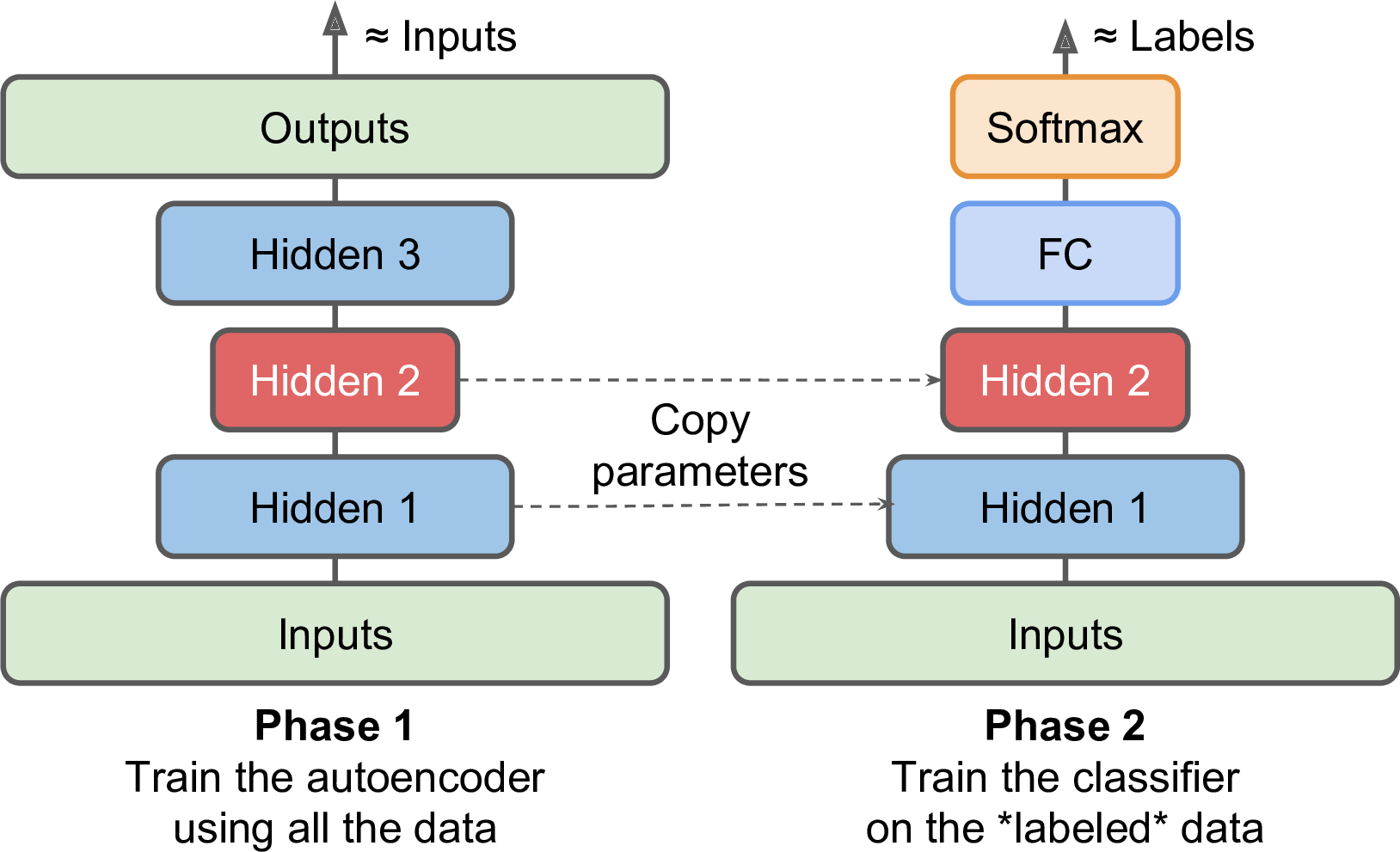## 15.5 去噪自编码器posted on 2018-05-29 15:15  royhoo  阅读(42332)  评论(4编辑  收藏